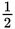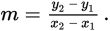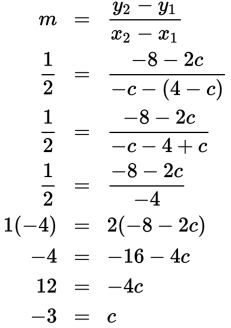# SAT Math Multiple Choice Question 556: Answer and Explanation

### Test Information

Question: 556

1. If a line that passes through the ordered pairs (4 – c, 2c) and (–c, –8) has a slope of, what is the value of c?

• A. –5
• B. –3
• C. –2
• D. 2

Explanation:

B

Difficulty: Medium

Category: Heart of Algebra / Linear Equations

Strategic Advice: Given two points (even when the coordinates are variables), the slope of the line between the points can be found using the formulaGetting to the Answer: You are given a numerical value for the slope and a pair of ordered pairs that have variables in them. To find the value of c, plug the points into the slope formula, and then solve for c. Be careful of all the negative signs.